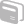# Activities

••• ##### Subject Area

• Math: Middle Grades Math: Algebraic Thinking
• Math: PreAlgebra: Linear Functions
• Math: Algebra I: Linear Functions

• ##### Author6-8

60 Minutes

• ##### Device
• TI-73 Explorer™
• TI-Navigator™

## Y-Intercept Exploration & Writing Equations of Lines

#### Activity Overview

Using TI-Navigator, students will discover the effect of 'b' on the graph of y = mx + b. Subsequently, they will learn to write the equation of a line in slope-intercept form.

#### Before the Activity

Students should have pre-requisite knowledge of y = kx graphs and have discussed slope to the point that y = mx graphs are well understood. Configure the Activity Center to allow students to send in one equation, let students view graphs of equations, and send current graph contents as background. Place the equation y = 2x + 3 on graph. Make sure you are on the 'Graph' tab so that the equation cannot be seen on the Activity Center screen.